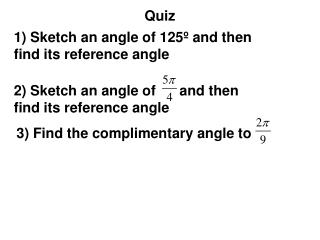# Quiz - PowerPoint PPT PresentationDownload PresentationQuiz

QuizDownload Presentation## Quiz

- - - - - - - - - - - - - - - - - - - - - - - - - - - E N D - - - - - - - - - - - - - - - - - - - - - - - - - - -
##### Presentation Transcript

1. Quiz 1) Sketch an angle of 125º and then find its reference angle 2) Sketch an angle of and then find its reference angle 3) Find the complimentary angle to

2. Quiz 1) Sketch an angle of 125º and then find its reference angle y 125º x Since it is 180º half way around the reference angle is 180 – 125 = 55º

3. Sketching Angles and Reference Angles 2) Sketch an angle of and then find its reference angle 5/4 = 1.2 2nd quadrant y x Since it is half way around the reference angle is

4. Quiz 3) Find the complimentary angle to

5. Finding sin, cos, and tan 1) Find the tan( ) This is not a special angle so we use the calculator – make sure it is in radians Round the answer to 4 decimal places

6. Inverse Trig Functions 1) Find the arctan( ) This question is asking us what angle has a tan = Look in our notes to find out that 30º has a tan =

7. Inverse Trig Functions 1) Find the arctan(.7638) This question is asking us what angle has a tan = .7638 Since this is not a special angle we use are calculator – 2nd tan to get:

8. Law of Sines Given a triangle with a combination of sides and angles we can use the law of sines to find the missing angles or sides A First we have to understand how to label the vertices and sides b c C The names of the vertices are given to you normally – the sides across from a vertex is labeled with a lower case letter a B

9. Law of Sines We use the law of sines ratio to find missing sides/angles A b We plug in the information that we are given and find what we are asked to find. c C a We will only use 2 parts at a time – not all 3 B

10. Law of Sines Find side c Now we cross multiply – make sure the calculator is in degree mode when taking the sin A b = 27ft c C B = 50º a C = 28º B

11. Law of Sines Find angle B Now we cross multiply A b = 12ft A = 42º c C a = 22ft Now we simply do 2nd sin (.3650) to get the angle B